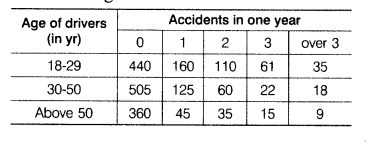# An insurance company selected 2000 drivers at random in a particular city

An insurance company selected 2000 drivers at random in a particular city to find a relationship between age and accidents. The data obtained are given in the following table.Find the probabilities of the following events for a driver chosen at random from the city
(i) being (18-29) yr of age and having exactly 3 accidents in one year.
(ii) being (30-50) yr of age and having one or more accidents in one year.
(iii) having no accident in one year.

Total number of drivers = 2000
∴ Total number of trials = 2000
(i) Let E1 be event of getting a driver being (18-29) yr of age and having exactly 3 accidents in a year. Then, number of trials in which E1 happened = 61
Hence,(ii) Let E2 be event of getting a driver being (30-50) yr of age and having one or more accidents in a year.
Then, number trials in which E2 happens
= 125+ 60 + 22 + 18 = 225
Now,P(E2)=225/2000=0.1125=0.113
(iii) Let E3 be event of getting a driver having no accident in a year.
Then, number of trials in which E3 happened
= 440+ 505+ 360 = 1305
∴ P (driver having no accident)
=1305/2000 = 0.6525 = 0.653